## Monte carlo Simulation for Adaptive Learning

Let us start with an example of a simple game. We want to make a game to improve the ability of user to memorize the . Conventional game without learning is basically showing the 48 characters randomly. The algorithm to show the syllable randomly is explained as follow.

To give equal chance to each syllable, we use random number generated by computer, which is also called a uniform distribution . Since it has 48 syllables and each syllable have equal chance, we say that the probability of each syllable would be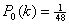. I use notation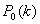to represent the probability distribution of each syllable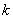. The probability distribution and cumulative probability distribution is shown in the table below.

 Syllables a u i e o ka ku ... ro wa o n Probability 1/48 1/48 1/48 1/48 1/48 1/48 1/48 1/48 1/48 1/48 1/48 Cumulative 1/48 2/48 3/48 4/48 5/48 6/48 7/48 45/48 46/48 47/48 48/48

To show the syllable with equal probability, we generate random number using computer and use the inverse cumulative distribution . The random number computer generate is between 0 and 1 inclusively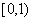. Suppose the random number generated by computer is denoted by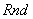, then

Show syllable a if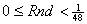Show syllable u if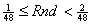Show syllable ka if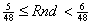Show syllable ku if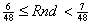Show syllable wa if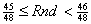Show syllable o if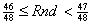Show syllable n if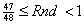The process to show the syllables randomly above is called Discrete Monte Carlo algorithm.